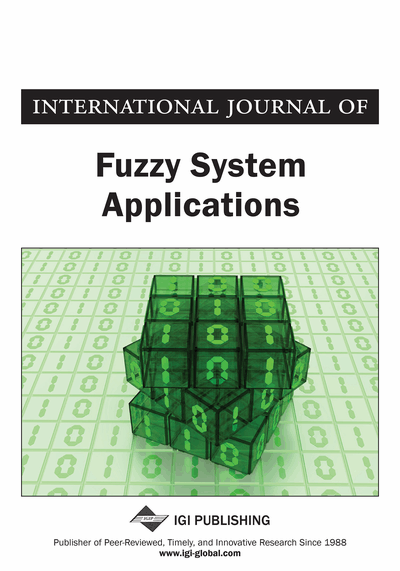# Interval-Valued Doubt Fuzzy Ideals in BCK-Algebras

Tripti Bej (Vidyasagar University, Midnapore, India) and Madhumangal Pal (Department of Applied Mathematics with Oceanology and Computer Programming, Vidyasagar University, Midnapore, India)
DOI: 10.4018/IJFSA.2019100106

## Abstract

Nearly forty years ago, interval-valued fuzzy sets were propounded by Zadeh as the normal ramification of fuzzy sets. This article focuses on the basics of a theory for such an interval-valued fuzzy set becoming interval-valued doubt fuzzy subalgebra and an interval-valued doubt fuzzy ideal of BCK-algebras. Also, the authors discuss fuzzy translation, fuzzy multiplication of an interval-valued doubt fuzzy subalgebra/ideal of a BCK-algebra. Besides this, the authors have attempted to substantiate a few common features relating them. At the same time, some properties of interval-valued doubt fuzzy ideals under homomorphism are investigated and the product of interval-valued doubt fuzzy ideals in BCK-algebras is also established.
Article Preview
Top

## Introduction

Fuzzy set was introduced by Zadeh (1965), as an extension of the classical notion of set. Literally, the word fuzzy often stands for the word vague. In the theory of fuzzy sets the membership degrees of elements range over the interval [0, 1]. The membership degree expresses the degree of belongingness of elements to a fuzzy set. The membership degrees in the interval (0, 1) denote the partial belongingness to a fuzzy set.

Again Zadeh (1975) made an extension of the concept of a fuzzy set by an interval-valued fuzzy set (i.e., a fuzzy set with an interval-valued membership function). This idea gives the simplest method to capture the imprecision of the membership grades for a fuzzy set. In this case the membership value of an element is not a single number, rather it is an interval and this interval is a subinterval of the interval [0, 1].

For the first time, in 1994, Biswas (1994) proposed the concept of Interval-valued fuzzy subgroups. After that in various algebraic structures, see (Jun, 2000; Senapati et al., 2012a, 2012b), the concept of interval-valued fuzzy sets have been studied.

The study of fuzzy algebraic structures was initiated by Rosenfeld in 1971, and thus opened a new direction, new exploration, new path of thinking to mathematicians, engineers, computer scientists, social scientists, physicists, chemists and many others. Since then a lot of important discrete structures have been fuzzified by many authors in various ways of various tests. It is true that not all of these fuzzifications have been seen in applications, but we are sure that many of these fuzzifications of algebraic structures will be nice tools for applications areas in due time.

The notion of BCK-algebras was propounded by Imai and Isꞌeki (1966a) in 1966. In the same year, Isꞌeki (1966b) introduced the notion of a BCI-algebra as a super class of BCK-algebras. Afterwards a vast study has been carried out investigating the algebraic properties of BCK/BCI-algebras and their relationship with other universal structures including lattices and Boolean algebras.

To develop the theory of BCK/BCI-algebras the ideal theory plays a pivotal role. In 1971, Rosenfield introduced the concept of fuzzy subgroup. Motivated by this, many mathematicians started to review various concepts and theorems of abstract algebra in the broader frame work of fuzzy settings. In 1991, Xi (1991) introduced the concept of fuzzy set to BCK-algebras. Then in 1992, Huang (1992) gave another concept of fuzzy set to BCI-algebras. Following the same rout Jun (1994) established the definition of doubt fuzzy subalgebra and doubt fuzzy ideal in BCK/BCI-algebras to avoid the confusion created in Huang’s (1992) definition of fuzzy BCI-algebras and gave some results about it. Several researchers investigated properties of fuzzy subalgebras and ideals in BCK/BCI-algebras see (Jun, 1993, 1994; Meng, 1994; Meng and Jun, 1994; Meng and Guo, 2005; Senapati et al., 2013, 2015; Bej and Pal, 2014, 2015a, 2015b, 2016).

Again, Jun (2000) introduced the notion of interval-valued fuzzy subalgebras/ideals in BCK-algebras and also the concept of fuzzy translation, fuzzy multiplication of fuzzy sub-algebras/ideals in BCK/BCI-algebras, has been discussed by Jun (2011) and Lee et al. (2009). After that in 2013, Senapati et al. (2013) extend this study from fuzzy translation to intuitionistic fuzzy translations in BCK/BCI–algebras. In the recent past Barbhuiya (2016) introduced fuzzy translations, fuzzy multiplications of interval-valued doubt fuzzy ideals of BF-algebras. Also, the authors (2014, 2015a, 2015b, 2016) have established several results on doubt intuitionistic fuzzy sub-algebras and ideals in BCK/BCI algebras.

## Complete Article List

Search this Journal:
Reset
Open Access Articles: Forthcoming
Volume 9: 4 Issues (2020): 1 Released, 3 Forthcoming
Volume 8: 4 Issues (2019)
Volume 7: 4 Issues (2018)
Volume 6: 4 Issues (2017)
Volume 5: 4 Issues (2016)
Volume 4: 4 Issues (2015)
Volume 3: 4 Issues (2013)
Volume 2: 4 Issues (2012)
Volume 1: 4 Issues (2011)
View Complete Journal Contents Listing